# KSEEB SSLC Class 10 Maths Solutions Chapter 2 Triangles Ex 2.2

In this chapter, we provide KSEEB SSLC Class 10 Maths Solutions Chapter 2 Triangles Ex 2.2 for English medium students, Which will very helpful for every student in their exams. Students can download the latest KSEEB SSLC Class 10 Maths Solutions Chapter 2 Triangles Ex 2.2 pdf, free KSEEB SSLC Class 10 Maths Solutions Chapter 2 Triangles Ex 2.2 book pdf download. Now you will get step by step solution to each question.

### Karnataka State Syllabus Class 10 Maths Chapter 2 Triangles Ex 2.2

Exercise 2.2 Class 10 Maths Triangles Question 1.
In the following fig. (i) and (ii). DE || BC.
Find EC in (i) and AD in (ii).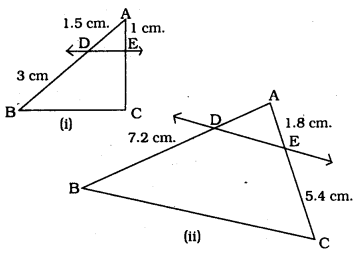Solution:
(i) In ∆ABC, DE || BC, EC =?
1.53=1EC
1.5 EC = 1 × 3
EC=31.5
EC=3015
∴ EC = 2 cm

(i) In ∆ABC, DE || BC, AD =?
=1.854×721
= 0.6 × 4

KSEEB Solutions For Class 10 Maths Triangles Question 2.
E and F are points on the sides PQ and PR respectively of a PQR For each of the following cases, state whether EF || QR:
(i) PE = 3.9cm. EQ = 3 cm
PF=3.6cm. FR = 2.4 cm.
(ii) PE = 4 cm. QE = 4.5 cm.
PF = 8 cm. RF = 9 cm.
(iii) PQ = 1.28 cm. PR = 2.56 cm.
PE = 0.18 cm. PF = 0.36 cm.
Solution: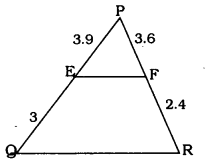In ∆PQR, if EF || QR then,
PEQR=PFFR
3.93=3.62.4
1.3 ≠ 1.5
Sides are not dividing In ratio.
∴ EF is not parallel to QR.

(ii)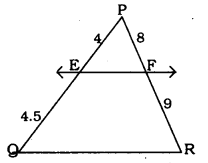In ∆PQR, if EF || QR then
PEQR=PFFR
44.5=89
89=89
Here sides are dividing in ratio.
∴ EF ||QR

(iii)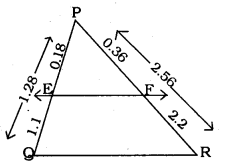PE + EQ = PQ
0.18 + EQ = 1.28
∴ EQ=1.28 – 0.18
EQ = 1.1 cm.
Similarly. PF + FR = PR
0.36 + FR = 2.56
FR = 2.56 – 0.36
FR = 2.2cm.
In ∆PQR, if EF || QR then
PEQE=PFFR
0.181.1=0.362.2
1.811=3.622
1.811=11.811
Here sides are dividing in ratio.
∴ EF || QR

10th Maths Triangles Exercise 2.2 Question 3.
In the following figure. If LM || CB and LN || CD, prove that AMAB=ANAD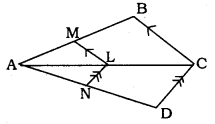Solution:
Data: LM || CB and LN || CD then prove that AMAB=ANAD
Solution: In ∆ACB, LM || CB
ALAC=AMAB……(1)( Theorem 1)
Similarly in ∆ADC, LN || CD
From equation (1) and (2) we have

Triangles Class 10 Exercise 2.2 Question 4.
In the following figure, DE ||AC and DF || AE. Prove that BFFE=BEEC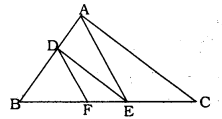Solution:
Data: In this figure, AE || AC and DF || AE, then we have to prove that BFFE=BEEC
Solution: In ∆ABC, DE || AC.
Similarly, In ∆ABE, DF ||AE.
from equation (1) and (2). we have
BEED=BFFE

Triangle Lesson Exercise 2.2 Question 5.
In the following figure. DE || OQ and DF || OR. Show that EF || QR.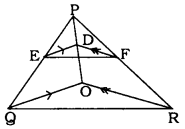Solution:
Data: In this figure. DE || OQ and DF || OR.
To Prove: EF || QR
Solution: In ∆POQ, DE || OQ.
PEEQ=PDDO…… (i) ( Theroem 1)
Similarly. In ∆POR. DF || OR.
PDDO=PFFR…… (ii) ( Theorem 1)
from equation (1) and (11), we have
PFEQ=PDDO=PFFR
PEEQ=PFFR
In ∆PQR. if PEEQ=PFFR then EF || QK. (∵ Theorem 1).

Triangles Class 10 Solutions KSEEB Question 6.
In the following figure, A, B an C are points on OP. OQ and OR respectively such that AB || PQ and AC || PR. Show that BC || QR.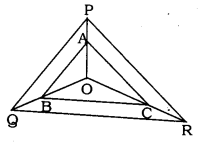Solution:
Data: In ∆QPR, AB || PQ and AC || PR. A, B, and C are the points on OP. OQ and OR.
To Prove: BC || QR
Solution: In ∆OPQ, AB || PQ.
OAAP=OBBQ…… (i) ( Theorem 1)
Similarly, In ∆OPR. AC|| PR.
OAAP=OCCR…… (i) ( Theorem 1)
from equation (i) and (ii), we have
OAAP=OBBQ=OCCR
OBBQ=OCCR
∴ BC || QR (∵ Theorem 2)

KSEEB Solutions For Class 10 Maths Question 7.
Using Theorem 2.1, prove that a line drawn through the mid-point of one side of a triangle parallel to another side bisects the third side. (Recall that you have proved it in Class IX).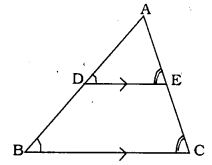Solution:
Data: In ∆ABC. D is the mid-point of AB.
DE Is drawn parallel to BC from D.
To Prove: DE bisects AC side at E.
∠D = ∠B (corresponding angles)
∠E = ∠C (corresponding angles)
12=AEAC
∴ AC = AE + EC
∴ E is the mid-point of AC
∴ DE bisects AC at E.

Triangles Class 10 KSEEB Solutions Question 8.
Using Theorem 2.2, prove that the line joining the mid-points of any two sides of a triangle Is parallel to the third side. (Recall that you have done it in Class IX)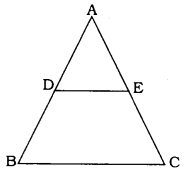Solution:
Solution: In ∆ABC, D and E are mid-points of AB and AC.
AE = EC
AC = 2AE
As per S.S.S. Postulate.
∴ They are equiangular triangles.
∴ ∠A is common.
These are pair of corresponding angles
∴ DE || BC

Triangles Class 10 Exercise 2.2 Solutions Question 9.
ABCD is a trapezium in which AB || DC and its diagonals intersect each other at the point O. Show that AOCO=CODO
Solution:
Data : ABCD is a trapezium in which AB || DC and its diagonals intersect each other at the point O.
To Prove: AOCO=CODO
Proof: In Trapezium ABCD. AB || DC.
∴ In ∆AOB and ∆DOC,
∠OCO = ∠OAB (alternate angles)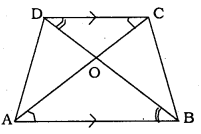∠ODC = ∠OBA (alternate angles)
∠DOC = ∠AOB (vertically opposite angles)
∴ ∆AOB and ∆DOC are equiangular triangles.
∴ ∆AOB ||| ∆DOC
Similar triangles divides sides in ratio.
AOCO=CODO

10th Maths Triangles Exercise 2.2 Solutions Question 10.
The diagonals of a quadrilateral ABCD intersect each other at the point O such that AOBO=CODO . Show that ABCD is a trapezium.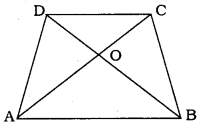Solution:
Data: In the quadrilateral ABCD. the diagonals intersect at 0’ such that AOBO=CODO
To Prove: ABCD is a trapezium.
Solution: In the qudrilateral ABCD, AOBO=CODO
AOCO=OBOD
It means sides of ∆AOB and ∆DOC divides proportionately.
∴ ∆AOB ||| ∆DOC.
Similarly. ∆AOD ||| ∆BOC.
Now, ∆AOB + ∆AOD = ∆BOC + ∆DOC
∆ABD = ∆ABC.
Both triangles are on the same base AB and between two pair of lines and equal in area.
∴ AB || DC.

All Chapter KSEEB Solutions For Class 10 Maths

—————————————————————————–

All Subject KSEEB Solutions For Class 10

*************************************************

I think you got complete solutions for this chapter. If You have any queries regarding this chapter, please comment on the below section our subject teacher will answer you. We tried our best to give complete solutions so you got good marks in your exam.

If these solutions have helped you, you can also share kseebsolutionsfor.com to your friends.

Best of Luck!!